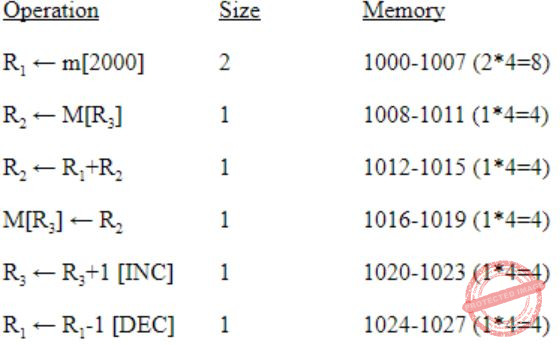October 8, 2023
October 9, 2023
October 8, 2023
###### Database-Management-System
October 9, 2023
 Question 119

Consider the following program segment. Here R1, R2 and R3 are the general purpose registers.

```       Instruction      Operation     Instruction size (no. of words)
MOV R1,(3000)   R1 ← m                2
LOOP: MOV R2,(R3)     R2 ← M[R3]                  1
ADD R2,R1       R2 ← R1 + R2                1
MOV (R3),R2     M[R3] ← R2                  1
INC R3          R3 ← R3 + 1                 1
DEC R1          R1 ← R1 - 1                 1
BNZ LOOP        Branch on not zero          2
HALT            Stop                        1 ```

Assume that the content of memory location 3000 is 10 and the content of the register R3 is 2000. The content of each of the memory locations from 2000 to 2010 is 100. The program is loaded from the memory location 1000. All the numbers are in decimal.

Assume that the memory is byte addressable and the word size is 32 bits. If an interrupt occurs during the execution of the instruction “INC R3”, what return address will be pushed on to the stack?

 A 1005 B 1020 C 1024 D 1040
Question 119 Explanation:
Memory is byte addressable, so it requires 1 word or 4 bytes to perform a each operation such that
→ Starts at memory location 1000.Interrupt occurs during the execution of information “INC R3”.

Then the value of address i.e., 1024 is pushed into the stack.
Question 119 Explanation:
Memory is byte addressable, so it requires 1 word or 4 bytes to perform a each operation such that
→ Starts at memory location 1000.Interrupt occurs during the execution of information “INC R3”.

Then the value of address i.e., 1024 is pushed into the stack.